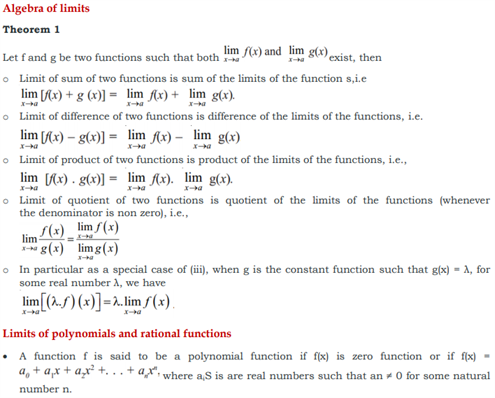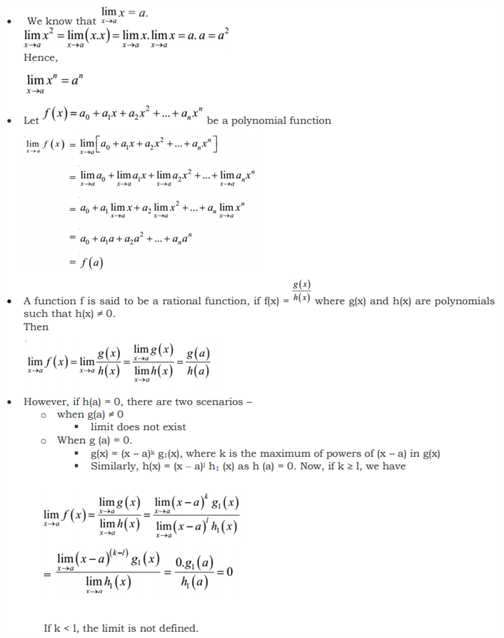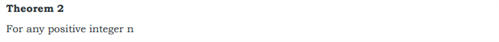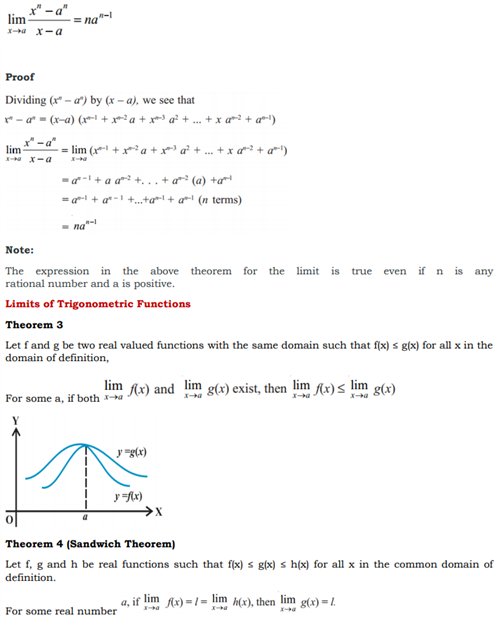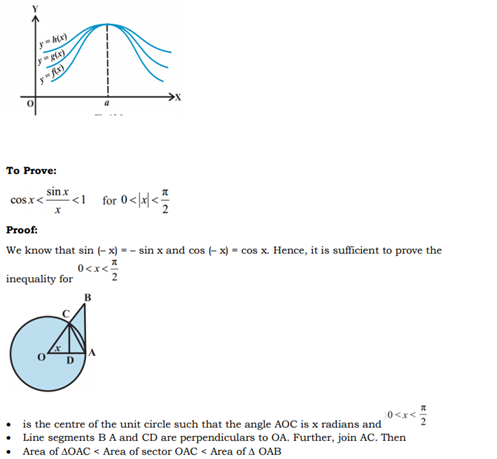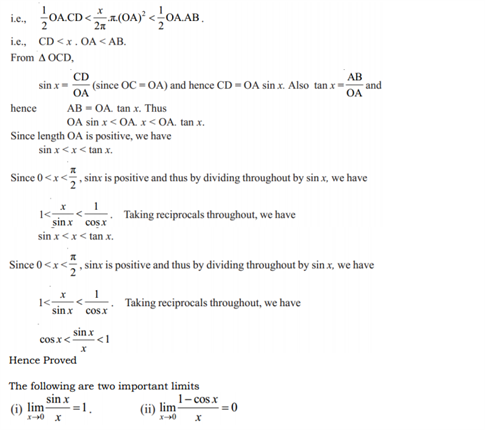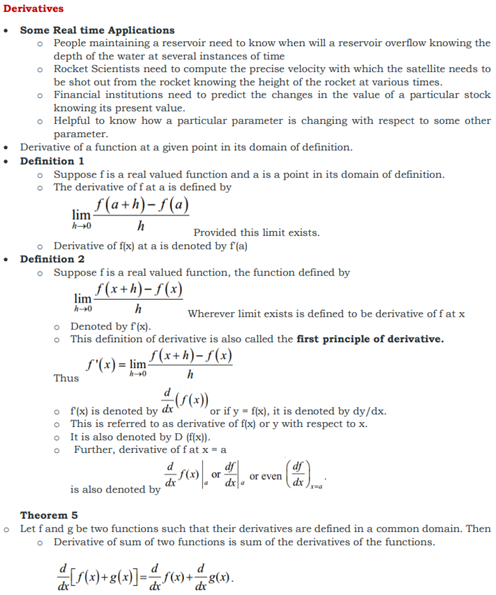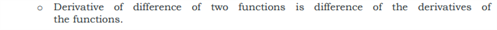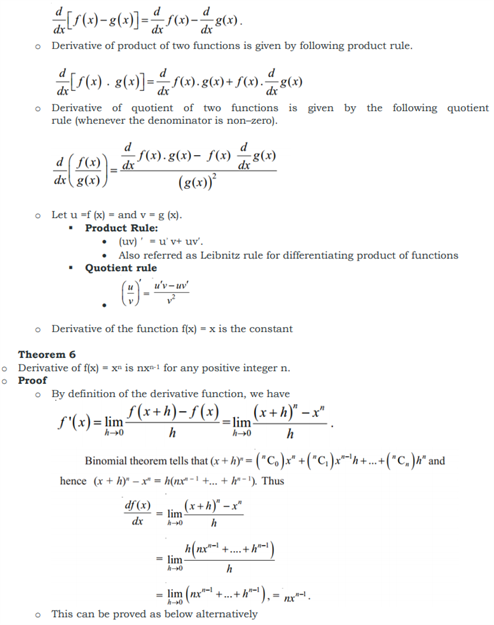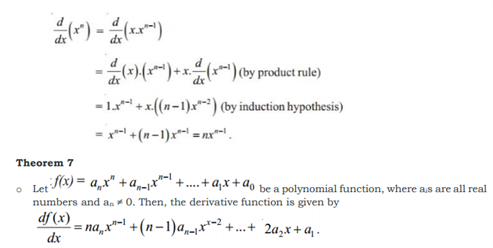# Revision Notes For CBSE Class 11 Maths Chapter 13 Limits and Derivatives

Class 11 maths revision notes chapter 13 Limits and Derivatives is carefully designed by subject experts. Calculus is that branch of mathematics which mainly deals with the study of change in the value of a function as the points in the domain change. We have provided the definition of the basic physical quantities used to represent limits such as :

• What is Limits?

In general as x → a, f(x) → l, then l is called limit of the function f(x) Symbolically written as For all the limits, function should assume at a given point x = a The two ways x could approach a number an either from left or from right, i.e., all the values of x near a could be less than a or could be greater than a.

The two types of limits Right-hand limit Value of f(x) which is dictated by values of f(x) when x tends to from the right. Left-hand limit. Value of f(x) which is dictated by values of f(x) when x tends to from the left. In this case the right and left hand limits are different, and hence we say that the limit of f(x) as x tends to zero does not exist (even though the function is defined at 0).

They would be prone to all the theorems in this chapter in maths. They would be witnessing limits of polynomial and rational functions. They would also be knowing the limits of trigonometric functions and derivatives etc.

Get here the Maths revision notes for class 11 chapter 13 Limits and Derivatives below.